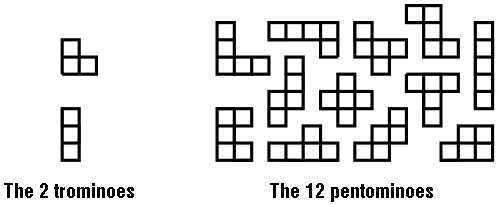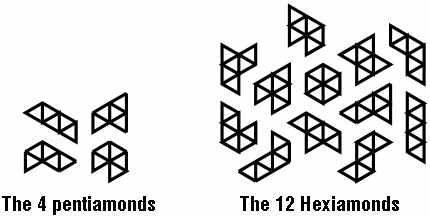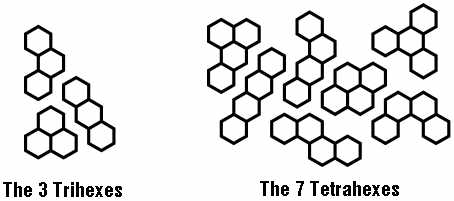Polyforms

Polyforms, literally “many forms,” are shapes that are built up from basic unit forms such as squares, triangles, hexagons, or circles.  Polyforms built of squares use the “-omino” ending.  There is only 1 domino, built of two squares, but there are 2 trominoes built of three squares, 5 tetrominoes built of four squares, 12 pentominoes built of five squares, 35 hexominoes built of six squares each, etc.“Polyiamonds” are built of unit equilateral triangles.  There are 4 pentiamonds built of five triangles and 12 different hexiamonds built of six triangles each.The “Polyhexes” are built of unit hexagons.  There are 3 trihexes built of three hexagons, 7 tetrahexes built of four hexagons each, 22 pentahexes built of five hexagons each, 82 hexahexes, etc.There are also Polytans (built of right triangles), Polyedges, Polycubes, etc.   For a more complete list of polyforms (and the numbers of each kind) see the World Game Review, issue #13, or check around the web.